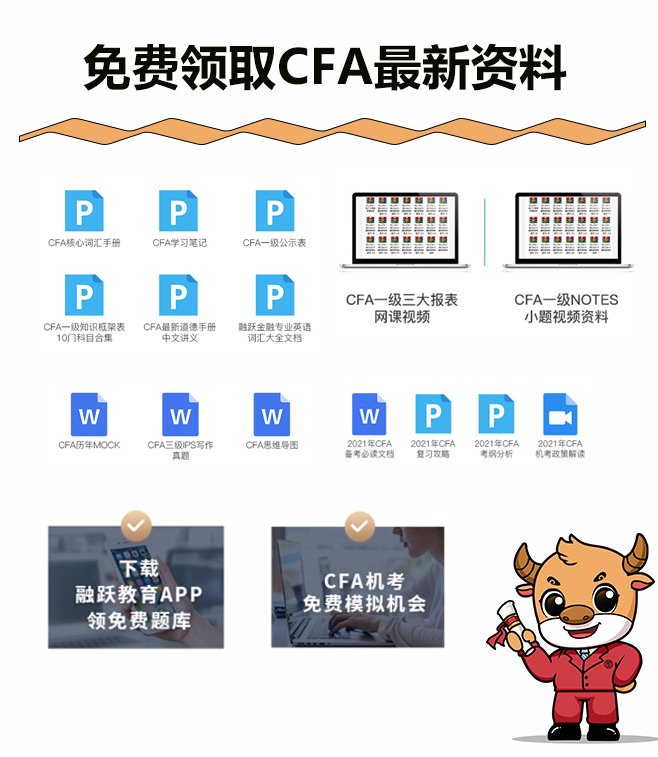Based on historical returns, a portfolio has a Sharpe ratio of 2.0. If the mean return to the portfolio is 20%, and the mean return to a risk-free asset is 4%, the standard deviation of return on the portfolio is closest to:

A．12%.

B．8%.

C．10%.

【答案】B查看答案

CFA备考怎么能少了CFA备考资料呢？小编为各位考生准备了CFA备考资料，有需要可以点击下方链接获取！【解析】

The Sharpe ratio for a portfolio p, based on historical returns, is defined as Where is the mean return to the portfolio is the mean return to a risk-free asset, and sp is the stancard deviation of return on the portfolio In this instance, 2= (20%-4%)/sp Solving forsp: sp = (20%- 4%)/2 = 8%